Compare Brokers

Vs.

# Free Forex Position Size Calculator

By Stelian Olar , Updated on: Nov 16 2023.

Welcome to your one-stop shop for everything related to Forex lot size calculator!

As an active Forex trader, you know how crucial it is to use proper position size Forex leverage and manage your risk. However, determining the right lot sizes in Forex can be confusing with all the varying Forex leverage and lot size options.

That's why I've created this handy web page with a free Forex lot size risk calculator for you to use. It takes into account your trading account size, risk tolerance, and stop loss distance to help you easily calculate Forex position size.

Whether you need to learn how to determine lot size in Forex for your next trade or want to learn more about calculating lot size Forex, you've come to the right place. I'll explain lot sizes in Forex in simple terms so you know exactly how to use leverage and position sizing together for smarter risk management.

Give the Forex calculator lot size a try and bookmark this page! You'll have an easy way to figure out the optimal positions for your Forex trading while protecting your account at the same time.

## What is Lot Size in Forex

If you're new to Forex trading, you may be wondering - what exactly is a "lot" and how does lot size work?

In simple terms, lot size refers to the number of currency units you buy or sell on each trade.

The base lot size in Forex is called a standard lot and a lot is 100,000 units of the base currency. So a standard lot of EUR/USD would be 100,000 euros.

Below the standard lots are:

• Mini lots - 10,000 units
• Micro lots - 1,000 units
• Nano lot - 100 units

The lot size directly impacts your profit potential and risk because it determines how much each pip movement is worth. For example, if you buy 1 standard lot (100,000 units) of EUR/USD at an exchange rate of 1.1000 and the rate increases by 10 pips to 1.1010, your trade would have made:

100,000 * 0.0010 (10 pips) = \$100 profit

As you can see, calculating position size properly using the right lot size is critical, especially when using leverage. That's where lot size Forex calculator come in handy to help determine the optimal size based on your account size, risk tolerance, and stop loss.

The key is finding the ideal balance - you want to maximize profits but without risking too much on each trade. Let's look at how to calculate lot size in Forex trading correctly.

## How To Use Our Position Size Calculator

Our free Forex position size calculator is designed to make determining the right trade size easy. Here's a step-by-step guide on how to use it:

### Select Instrument

First, choose the currency pair or instrument you want to trade - Forex, stocks, futures, cryptocurrencies, etc. The calculator will be customized for that instrument (e.g. futures position size calculator). On the position size calculator, select the currency pair you want to trade such as EUR/USD or USD/JPY.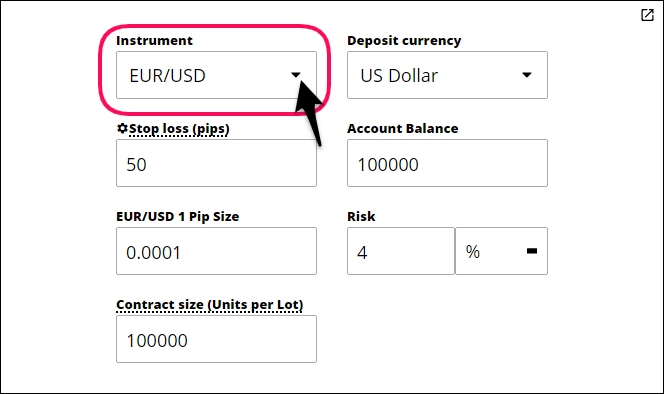For example, if you want to trade the EUR/USD pair, you would select "EUR/USD" from the instrument dropdown menu.

Note* If you’re a stock trader, you can use it as a stock position size calculator.

### Select Deposit Currency

Next, choose the account currency your trading account is denominated in - typically USD, EUR, GBP, etc. The deposit currency helps convert the pip value for position sizing calculations.

For example, if your account is in US dollars, you would select "USD" as the deposit currency.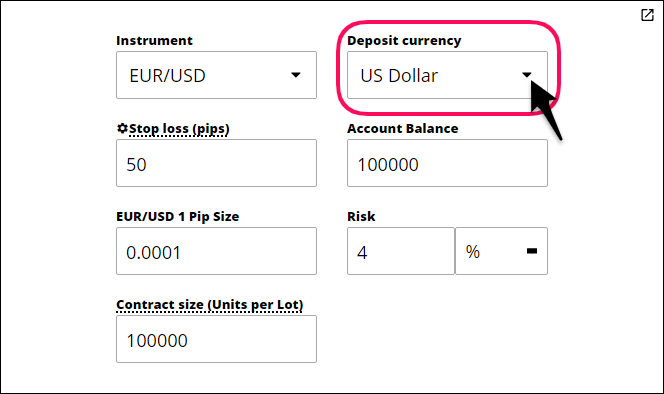### Set Stop Loss Price

Determine the stop loss price where you will exit the losing trade. Enter this stop loss price.

You may set a 50 pip stop loss at 1.1200. So you would input the number of pips ie 50 pips as the stop loss price.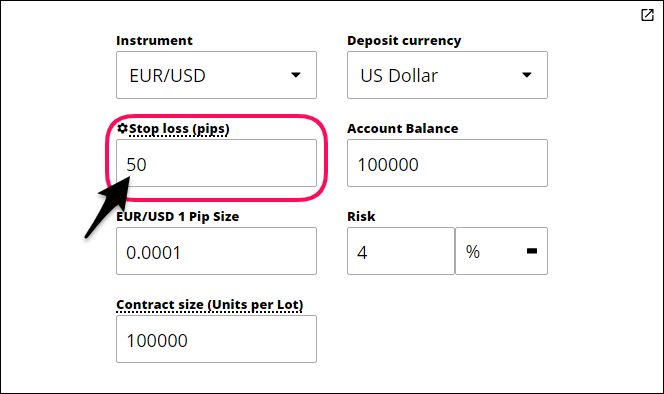### Enter Account Size

Input the total value of your Forex trading account balance. This is used by the stock position size calculator to determine the proper position size based on the percent risk you are willing to take.

Let's say your account size is \$10,000. You would enter 10,000 into the account size field.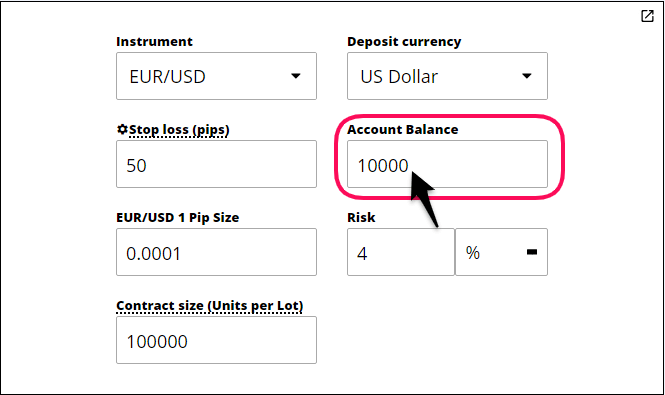### Set Risk Percentage

Decide how much of your account to risk on the trade. The 2% rule is recommended, but you can choose any amount, often between 1-5%.

For example, you may choose to risk 2% of your account per trade. So for your \$10,000 account size, you would enter 2% as the risk percentage.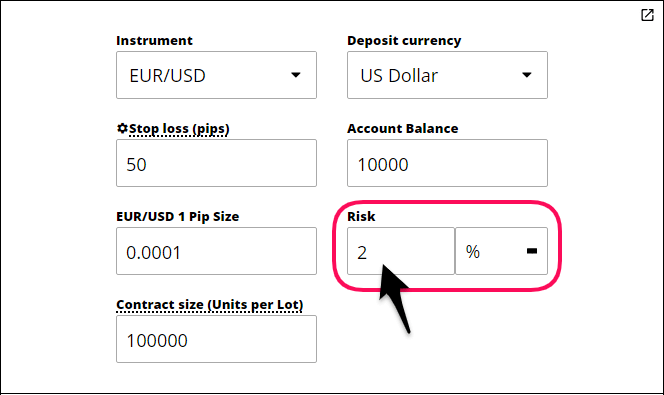### Click Calculate and Review Position Sizing Results

Review all inputs to ensure they are correct and then click calculate to determine the ideal position size. The position size calculator MT5 will show the maximum position size based on your inputs.

It may recommend a position size of 0.4 standard lots (40,000 US dollars) based on a 2% risk of your \$10,000 account, which translates into \$200 money at risk.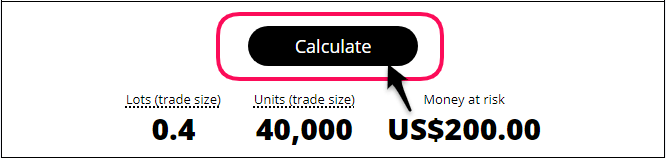Tweak inputs like risk percentage and stop loss to get position sizing that meets your risk tolerance. Always double-check the position size calculator MT4 results to make sure you’re comfortable trading with the recommended trade size and lower the risk percentage to re-calculate if the trade size is “too big.”

## What is the Formula for Position Size Trading?

Now that you know what lot size is, let's look at the actual Forex lot size formula to use when you calculate position size.

The basic formula to calculate lot size Forex is:

(Account Size * Risk %) / (Entry Price - Stop Loss) = Lot Size

For example, if you have a \$10,000 account balance, and are willing to risk 2% per trade (\$200), with a 30 pip stop loss on a EUR/USD trade entered at 1.1500, then here is how to determine lot size Forex:

(\$10,000 * 0.02) / (1.1500 - 1.1470) = 0.2 standard lots

So you would trade 0.2 standard lots or 20,000 units of EUR/USD to adhere to your 2% risk with proper position sizing.

This formula allows you to determine the optimal position size based on the amounts of money you have in your trading account, acceptable risk level, entry price and stop loss distance.

Rather than manually plugging in the variables each time, I recommend using an online Forex trading lot size calculator or position size calculator Forex to simplify the process.

The key is consistency - always use the same Forex position size calculator and inputs to determine the right position size for your trading system and account size. This helps manage risks and maximize profits in the long run.

## How Do You Set Position Size?

Now that you know the formula, let's discuss some tips for actually setting your [position size] in forex trading:

1. Start small - When in doubt, trade smaller than your maximum calculated position size. You can always increase your lot size later once you are more comfortable.
2. Consider volatility - Highly volatile currency pairs like GBP/JPY require smaller position sizes than slower pairs like EUR/CHF. Use a lower risk % for volatile pairs.
3. Factor in free margin - Check your available free margin before placing trades to reduce the chances of a margin call. Adjust your lot size if you have a limited free margin and many open positions.
4. Use a Forex standard lot size calculator - Input your account size, risk %, stop loss, and currency pair into a Fx position size calculator to simplify the math.
5. Be consistent - Stick to the same inputs and calculate position size Forex every time to correctly size all your positions.

Proper position sizing takes some practice but is key for managing risks. Start small and use a Forex calculator lot size with leverage until you get the hang of setting appropriate lot sizes for your trades and account balance. This will help you survive long-term and avoid margin call headaches.

Want to put these Forex position sizing tips into practice?